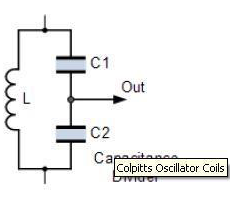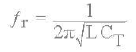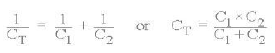Courses

# The Colpitts Oscillator Electrical Engineering (EE) Notes | EduRev

## Electronic Devices

Created by: Cstoppers Instructors

## Electrical Engineering (EE) : The Colpitts Oscillator Electrical Engineering (EE) Notes | EduRev

The document The Colpitts Oscillator Electrical Engineering (EE) Notes | EduRev is a part of the Electrical Engineering (EE) Course Electronic Devices.
All you need of Electrical Engineering (EE) at this link: Electrical Engineering (EE)

The Colpitts Oscillator

The Colpitts Oscillator, named after its inventor Edwin Colpitts is another t ype of LC oscillator  design. In man y wa ys, the Colpitts oscillator is the exact opposite of the Hartley Oscillator we looked at in the previous tutorial. Just like the Hartley oscillator, the tuned tank circuit consists of an LC resonance sub- circuit connected between the collector and the base of a single stage transistor amplifier producing a sinusoidal output waveform.

The basic configuration of the Colpitts Oscillator resembles that of the Hartley Oscillator but the difference this time is that the centre tapping of the tank sub- circuit is now made at the junction of a "capacitive voltage divider"  network instead of a tapped autotransformer t ype inductor as in the Hartley oscillator.The Colpitts oscillator

The Colpitts oscillator uses a capacitor voltage divider as its feedback source.
The two capacitors, C1 and C2 are placed across a common inductor, L as shown so that C1, C2 and L forms the tuned tank circuit the same as for the Hartley oscillator circuit.

The advantage of  this t ype of tank circuit configuration is that with less self and mutual inductance in the tank circuit, frequency  stability is improved along with a more simple design. As with the Hartley oscillator, the Colpitts oscillator uses   a single stage bipolar transistor amplifier as the gain element which produces a sinusoidal output. Consider the circuit below.

Basic Colpitts Oscillator Circuit

The transistor amplifiers emitter is connected to the junction of capacitors, C1 and C2 which are connected in series and act as   a simple voltage divider. When the power supply is firstl y applied, capacitors C1 and C2 charge up and then discharge through the coil L. The oscillations across the capacitors are applied  to  the base- emitter junction and appear in the amplified at the collector output. The amount of feedback depends on the values of C1 and C2 with the smaller the values of C the greater will be the feedback.

The required external phase shift is obtained in a similar manner to that in the Hartle y   oscillator circuit with the required positive feedback   obtained for sustained un- damped oscillations.  The amount of feedback is determined by the ratio of C1 and C2 which are generall y "ganged" together to provide a constant amount of feedback so as one is adjusted the other automaticall y follows.

The frequenc y of oscillations for a Colpitts oscillator is determined b y the resonant frequenc y of the LC tank circuit and is given as:where CT is the capacitance of C1 and C2 connected in series and is given as:.The configuration of the transistor amplifier is of a Common Emitter Amplifier with the output signal 180 o out  of phase with regards to the input signal. The additional 180 o phase shift require for oscillation is achieved b y the fact that the two capacitors are connected together in series but in parallel with the inductive coil resulting in overall phase shift of the circuit being zero or 360o .

Resistors, R1 and R2 provide the usual stabilizing DC bias for the transistor in the normal manner while the capacitor acts as a DC- blocking capacitors. The radio- frequency choke (RFC) is used to provide a high reactance (ideally open circuit) at the frequency of oscillation, ( ƒr ) and a low resistance at DC.

Colpitts Oscillator using an Op- amp

As well as using a bipolar junction transistor (BJT) as the amplifiers active stage of the Colpitts oscillator, we can also use either a field effect transistor, (FET) or an operational amplifier, (op- amp). The operation of an Op- amp Colpitts Oscillator is exactly the same as for the transistorised version with the frequency of operation calculated in the same manner. Consider the circuit below.

Colpitts Oscillator Op- amp Circuit

The advantages of the Colpitts Oscillatorover the Hartley oscillators are that the Colpitts oscillator produces a more purer sinusoidal waveform due to the low impedance  paths of the capacitors at high frequencies. Also due  to these capacitive reactance properties the Colpitts oscillator can operate at very high frequencies into the microwave region.

WIEN BRIDGE OSCILLATOR

One of the simplest sine wave oscillators which uses a RC network in place of the conventional LC tuned tank circuit to produce a sinusoidal output waveform, is the Wien Bridge Oscillator.

The Wien Bridge Oscillator is so called because the circuit is based on a frequenc y- selective form of the Whetstone bridge circuit. The Wien Bridge  oscillator is a two- stage RC coupled amplifier circuit that has good stabilit y at its resonant frequency, low distortion and is very eas y to tune making it a popular circuit as an audio frequency oscillator

Offer running on EduRev: Apply code STAYHOME200 to get INR 200 off on our premium plan EduRev Infinity!

,

,

,

,

,

,

,

,

,

,

,

,

,

,

,

,

,

,

,

,

,

;